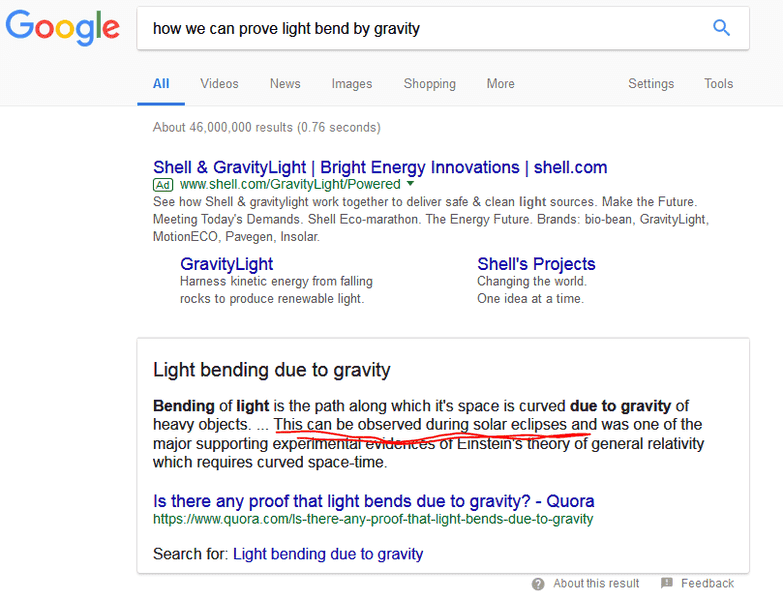# Principle of equivalence

Consider 2 lifts ,one on ground and other on acceleration, principle of equivalance says you can't find you are on a gravitational field, or accelerating. g decrease when r increase so I can find which lift on gravitational field ?

Ibix
Of course. The equivalence principle, strictly speaking, only applies at a point (any point). It applies approximately over a small region, but over a large enough region curvature becomes apparent.

Of course. The equivalence principle, strictly speaking, only applies at a point (any point). It applies approximately over a small region, but over a large enough region curvature becomes apparent.
If it is a point then how lift experiment could possible,

And how we can prove light bend by gravity

Ibix
If it is a point then how lift experiment could possible,
Because the lift is a small region and the equivalence principle applies approximately. Calculate the different (Newtonian) accelerations at the bottom and top of a typical lift. Could you detect that difference?

Formally, the equivalence principle means that the second derivatives of the metric can be made to vanish at a chosen point by an appropriate choice of coordinates, and the smoothness of the metric means that the second derivatives are small nearby. So no matter how precise your experiment, there's always a smaller separation where you will not be able to detect a difference between being on a rocket and on a planet.

•Dale
Ibix
And how we can prove light bend by gravity
Google for Eddington's measurements during a solar eclipse.

Nugatory
Mentor
And how we can prove light bend by gravity
Eddington, as @Ibix says, and also observations of gravitational lensing.

phinds